# Fractions + area of shape - math problems

Area is the quantity that expresses the extent of a two-dimensional shape. The area can be understood as the amount of paint necessary to cover the surface with a single coat. The area of a shape can be measured by comparing the shape to squares of a fixed size 1 m^2 or 1 cm^2 etc. Every unit of length has a corresponding unit of area. Areas can be measured in square metres (m^2), square centimetres (cm^2), square millimetres (mm^2), square kilometres (km^2), square feet (ft^2), square yards (yd^2), square miles (mi^2), and so forth.

#### Number of problems found: 31

• In theIn the national park, the ratio of the wooded area to grassland is 4: 1. The total area is 385km2. What area is wooded?
• DivideDivide area of rectangles with dimensions 32m and 10m by the ratio 7: 9. What area corresponds to a smaller section?
• Garden exchangeThe garden has the shape of a rectangular trapezoid, the bases of which have dimensions of 60 m and 30 m and a vertical arm of 40 m. The owner exchanged this garden for a parallelogram, the area of which is 7/9 of the area of a trapezoidal garden. What is
• Length 7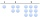Length equals 7/6 inch in width equals 7/9 inches the area is?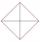In the square ABCD point P is in the middle of the DC side and point Q in the middle pages AD. If the area of quadrilateral BQPC is 49 cm2, what is the area of ABCD?
• GardensThe area of the square garden is 3/4 of the area of the triangular garden with sides of 80 m, 50 m, 50 m. How many meters of the fence do we need to fence a square garden?
• MO Z9–I–2 - 2017In the VODY trapezoid, VO is a longer base and the diagonal intersection K divides the VD line in a 3:2 ratio. The area of the KOV triangle is 13.5 cm2. Find the area of the entire trapezoid.
• Garden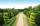The garden has a rectangular shape with lengths of 25 and 40 meters. It has been expanded so that each of its size increased by one fifth. How many square meters increased its acreage?
• Hamster cage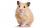Ryan keeps his hamster cage on his dresser. The area of the top of Ryan's dresser is 1 2\3 as large as the area of the bottom of his hamster cage. The area of the dresser top is 960 square inches. How many square inches of his dresser top are not covered
• Irrigation sprinklerThe irrigation sprinkler can twist with an angle of 320° and has a reach of 12 meters. Which area can irrigate?
• Three segments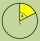The circle is divided into 3 segments. Segment A occupies 1/4 of the area, segment B occupies 1/3 of the area. What part is occupied by section C? In what proportion are areas A: B: C?
• Garden GThe rectangular trapezoidal garden has a base length of 81m, 76m, and a vertical arm of 12m. Calculate how many m2 of area will remain for planting greenery, if 1/3 of the area is built. Calculate the consumption of mesh for land fencing.
• Cuboid boxHow many m2 paper is needed for the sticking cuboid box of dimensions 50 cm, 40 cm and 30 cm? To the folds add one-tenth the area.
• Three shapes1/5 of a circle is shaded. The ratio of area if square to the sum of area of rectangle and that of the circle is 1:2. 60% of the square is shaded and 1/3 of the rectangle is shaded. What is the ratio of the area of circle to that of the rectangle?
• FloorThe floor area of ​​the room is 31 m2 and has a width of 4.3 m. How many centimeters of circumference measured floor on the map at the scale 1:75?
• Trapezoid MOThe rectangular trapezoid ABCD with the right angle at point B, |AC| = 12, |CD| = 8, diagonals are perpendicular to each other. Calculate the perimeter and area of ​​the trapezoid.
• Right triangle from axesA line segment has its ends on the coordinate axes and forms with them a triangle of area equal to 36 square units. The segment passes through the point ( 5,2). What is the slope of the line segment?
• Mice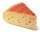Mice consumed a circular hole in a slice of cheese. The cheese has the shape of a circular cut with a radius of 20 cm and an angle of 90 degrees. What percentage of the cheese ate mice if they made 20 holes with a diameter of 2 cm?
• Trapezoid thirdsThe ABCD trapezoid with the parallel sides of the AB and the CD and the E point of the AB side. The segment DE divides the trapezoid into two parts with the same area. Find the length of the AE line segment.
• Cutting square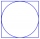From a square with a side of 30 cm, we cut the circle with the highest possible diameter. How many percents of the square content is this circle?

Do you have an interesting mathematical word problem that you can't solve it? Submit a math problem, and we can try to solve it.

We will send a solution to your e-mail address. Solved examples are also published here. Please enter the e-mail correctly and check whether you don't have a full mailbox.

Please do not submit problems from current active competitions such as Mathematical Olympiad, correspondence seminars etc...